# Playing around with Routing Tables

Task: Create a network Topology Setup in such a way so that System A can ping to two Systems, System B and System C but both these systems should
not be pinging each other without using any security rule e.g. firewall etc.

Since we aren’t allowed to take the help of firewalls, we’ll have to search for ways to either masquerade our IPs’, disrupt the connection between systems without actually touching the hardware (firewall would have been a great help with this), or make the IPs’ unreachable to each other. One such way to do just that, i.e. make IPs’ unreachable to each other, is through routing table.

Routing table: Routing table or Routing Information Table is a table stored in a router or a network host that lists the routes to particular network destinations, and in some cases, metrics associated with those routes.

Now to manipulate or control the routing table in Linux, we have command `route` which we can use to add or delete routes to required destination IP addresses. Hence we have all the tools in our arsenal to complete the task, so let get started…

To do this task I’ve summoned three RHEL8 VMs (2 CLI for making our system B and system C and a GUI for being our system A). The first thing we’ll need to do is think about how to go about creating our routing table so that it fulfills our requirement. The first idea would be to make the routing table of system A as big as possible, such that system B and system C are both covered by it.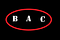Let the letters represent the systems respective to system names. Red ring represents routing table of system A

Now since we’ll also need to receive replies back for our pings for system A, so the routing tables of B and C must cover system A but not each other.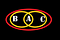The yellow rings represent routing tables of system B and system C

Now understanding this in terms of numbers, one thing’s of sure system A should be in the middle of system B and system C in terms of IP, why? Since, the systems itself must be covered by it’s own routing table, or at least the IP that will be used to store the rule must be under this it. And since B and C wants to ping A but not each other, the smallest range for it must be from A to the respective system. But if say A comes before B then the routing table of C will also engulf system B’s IP in its range and then though we’ll not get replies back from B, network packets can be sent to it. So let’s say system A is IP 2, system B is 1 and system C is IP 3, i.e. x.y.z.1, x.y.z.2 and x.y.z.3, where x, y and z for all three of the systems are same. Again why? Well, it’s so to make all three of the systems to be the part of a single network so that we don’t have to deal with the router.

Now, at this point we will also have to take in account the IPs which are pre-consumed or will have to be consumed for our system to manage the routing table for example IP x.y.z.0 will be consumed to store the network range in the routing table given that all three systems store the same single rule. Also there will be another IP at the end of the range which will store the broadcast IP of the range(part of a big network:- subnet). Hence whenever create a rule i.e. we give a range for which our network operations will take place, i.e. whenever we create a subnet 2 IPs will be consumed to store:

1. Our range (Network Name). Comes at the extreme beginning of the range.
2. our broadcast IP. Comes at the extreme end of the range.

Also note that these IPs can be shared by multiple subnets, but one broadcast cannot be another subnet’s Network Name. Hence we will need to change IPs for system A and C (B can still be IP 1). So let’s say A and B share network name (x.y.z.0) and A and C share broadcast IP, then C’s network name has to be placed before A, and B’s broadcast has to be placed in between A and C. So again the smallest way to do this will be to give C network name as 2, and so A can be 3 after which we can keep the broad IP of B at 4 and hence C can have IP 5 and then A and C will have shared broadcast at IP 6.

Now to understand we can imagine containers which exist in this realm of networking and whose side-walls are 1 IP thick, and whose color decides who own them, and they can also somehow intersect each others’ space. A visual representation of this is tried to be shown below…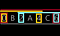Yellow represents container for B, aqua for C and maroon for A

Now here the lid of the container represents the rule we’ll have to add for the respective system. For example for A it is x.y.z.0 to x.y.z.6. However we usually do not give ranges like this in networking, we provide ranges in terms of where to start and how long to go from there. So for A it will be start at x.y.z.0 and we need stop at 6, i.e. we need a total of 7 IPs in A’s subnet. And to say we need 7 IP, we will need to tell the netmask(Genmask) to the system.

Netmask: A Netmask is a 32-bit “mask” used to divide an IP address into subnets and specify the network’s available hosts. In a netmask, two bits are always automatically assigned.

Hence for our example of A, the netmask will be 255.255.255.256IPs–7IPs=255.255.255.249. Now let’s say if we wanted to allocate 300 IPs then our netmask will be 255.255.255.256–300=255.255.254.226. And so on… For someone wondering as to what these numbers mean, each section of these IPs represent 1 byte, i.e. 8 bits, 8 1s and 0s i.e. the way a normal computer work. Hence 255.255.255.255 actually is 11111111.11111111.11111111.11111111. And since the total possibility per bit is 2, and 2⁸ is 256, but in computer things start from zero unlike us counting in natural numbers, and hence 256 is counted as 0,1,2,3…255. And the 256 is converted to natural number for calculation purpose. Hence a netmask will tell how many bits can be changed inside a subnet to produce a IP in that subnet. i.e. for A the bits that be changed are 256–249=7 converted to binary=111 hence we can change the last 3 bits of the IP, however if we look into it, we can see that if we have a wiggle room of 3 bits we can fit 8 IPs and so to have room for exactly 7 IPs we have to restrict our system to take one of the IP from the range, which will create a lots of mess. Hence to overcome this the IP and subnets are designed such as so they are convenient to use, instead of making the user do all the binary math.

Hence the netmask can only be created only when all the 1s in the starting of the string are continuous i.e. 11111111.11111111.11111111.10000000 is feasible but something like 11111111.11111111.11111111.11111001 is not. Also if we perform ‘bit-wise-and’ between some IP and the subnet for that we will get our Network Name. This restricting of subnet also leads us to another way to represent subnet, i.e. through prefix-length which is equal to number of 1s that we have in in the subnet. And hence an IP with its subnet can be written as IP/prefix-length as a short-hand.

The restriction can be understood by our container model as:- there are restriction to what our container’s size can be. The size restrictions are put such that the size of the container can be 2^n only, where ’n’ is a whole number however since 2 IPs will be consumed it only makes sense to use n>1. Also so to make the subnet division as efficient as possible, the network name of any subnet is fixed(based on the length, the only length that can access all IP as network name is 0), and hence the same translates to the broadcast IP.

Which means, that we cannot have intersecting containers, they can only share walls. Also since broadcast and network name cannot be on the same IP, hence all the equally sized containers will always be placed such that they start at a.b.c.0 and end at a.b.c.255, or on a bigger scale, they’ll be placed side-by-side from 0.0.0.0 to 255.255.255.255.

So, finally what does this mean for us? That we can’t have overlapping IPs of same size. Also when we add up some of these to form up a bigger container, we can only add such that the rule of 2^n is not broken and hence we can only add up containers in number of again 2^m.

And hence there is no way we can have overlapping subnets, the subnets can only be engulfed by the bigger subnet. And hence the best solution for this task is to make A and some other system have same subnet and make a rule to let systems in the that subnet be able to ping each other.

So the practical for this will go something like as follows:-

Here we first set(change) the IP of system A as 192.168.1.5, and since we want it’s subnet(the subnet which contains A) has 8(minus 2) systems, as the only smaller range is 4(minus 2 = 2 IPs) which is inadequate for our working, our prefix length comes out to be 29 (8=2³; 32–3=29). This was done with the command `ipconfig enp0s3 192.168.1.5/29` , then we deleted all the pre-existing rules using `route del -net 192.168.122.0/24` and added our new rule which will allow A to connect all over its subnet, by using the command `route add -net 192.168.1.0 netmask 255.255.255.248 enp0s3` , after which we printed the routing table using the command `route -n` . And at last we checked the IP of the system of the system using the command `ipconfig enp0s3` and as we can see in the last line the inet is our new IP.

Now we must do the same for our other systems so that they can reply back to system A. We picked IP of B as 192.168.1.1 and system C as 192.168.1.6, where subnet of A and B is the same and ingulfs subnet of C.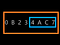The orange container(subnet) is for A and B while the blue one is for C

Hence now A can ping both B and C, and though B can send packets to C, it won’t get any replies as C can’t make it’s packet reach B. The practical implementation of this and the ping test for this looks something as follows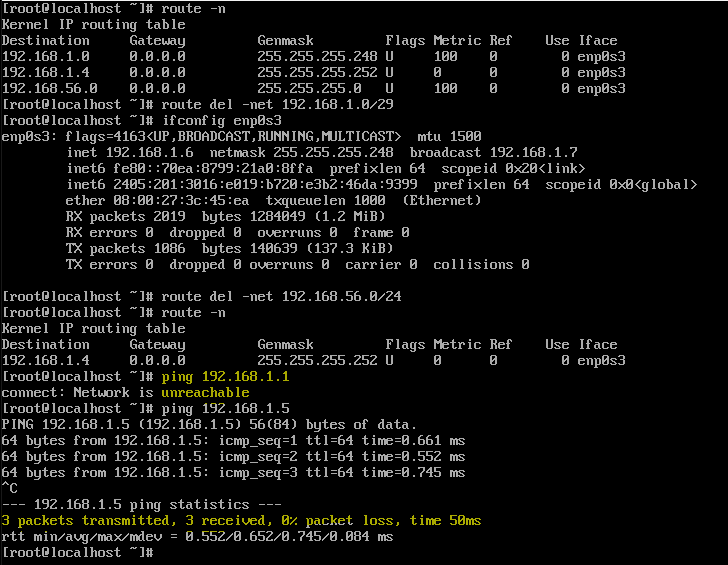First snippet shows system B and second snippet shows system C

We can see that ping for system A is successful with 0% packet loss, while with the other system the packet loss is either 100% (From system B) or is either said to be unreachable (From system C), hence the latter part of the task is done.

Now Finally let’s see if A is able to ping both B and C, so to see if our task is completed…

So we can see that we have 0% loss for pings to both the system B and system C respectively.

That’s it…